## Symmetrical Component Transformation:

Symmetrical Component Transformation is given by a  set of three balanced voltages (phasors) Va,Vb,Vc is characterized by equal magnitudes and interphase differences of 120°. The set is said to have a phase sequence abc (positive sequence) if Vb lags Va by 120° and Vc lags Vb by 120°.

The three phasors can then be expressed in terms of the reference phasor Va as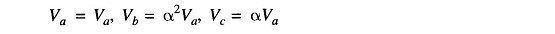where the complex number operator α is defined as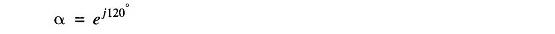It has the following properties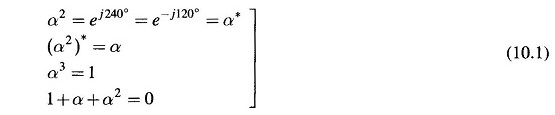If the phase sequence is acb (negative sequence), then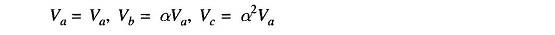Thus a set of balanced phasors is fully characterized by its reference phasor (say Va) and its phase sequence (positive or negative).

Suffix 1 is commonly used to indicate positive sequence. A set of (balanced) positive sequence phasors is written as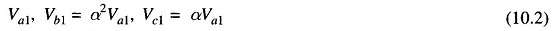Similarly, suffix 2 is used to indicate negative sequence. A set of (balanced) negative sequence phasors is written as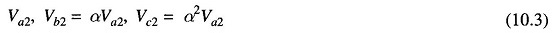A set of three voltages (phasors) equal in magnitude and having the same phase is said to have zero sequence. Thus a set of zero sequence phasors is written as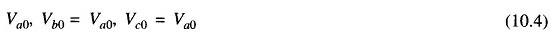Consider now a set of three voltages (phasors) Va,Vb,Vc which in general may be unbalanced. According to Fortesque’s theorem the three phasors can be expressed as the sum of positive, negative and zero sequence phasors defined above. Thus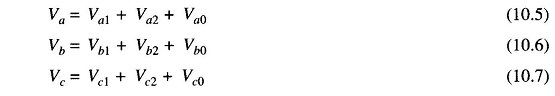The three phasor sequences (positive; negative and zero) are called the symmetrical component transformation of the original phasor set Va,Vb,Vc The addition of symmetrical component transformation as per Eqs. (10.5) to (10.7) to generate Va,Vb,Vc is indicated by the phasor diagram of Fig. 10.1.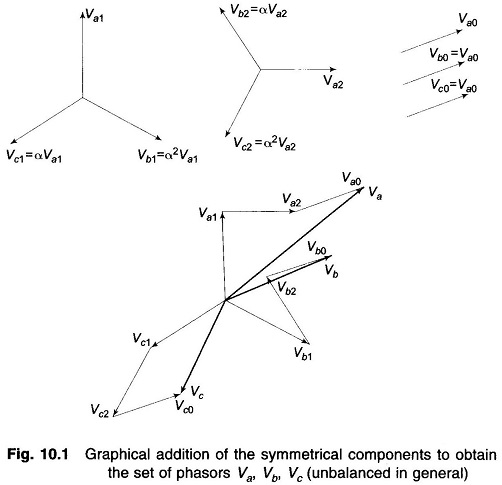Let us now express Eqs. (10.5) to (10.7) in terms of reference phasors Va1, Va2 and Va0. Thus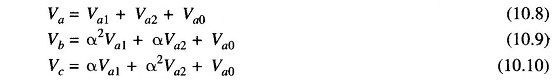These equations can be expressed in the matrix form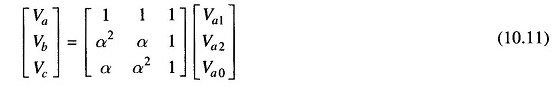or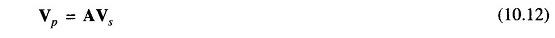Where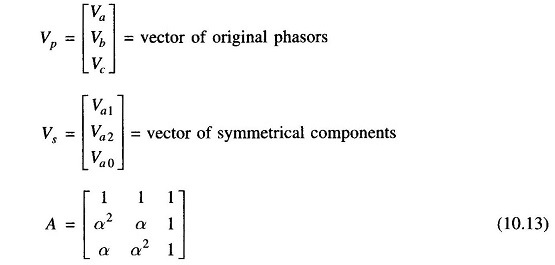We can write Eq.(10.12) as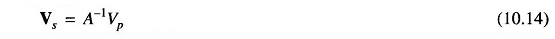Computing A-1 and utilizing relations (10.1) , we get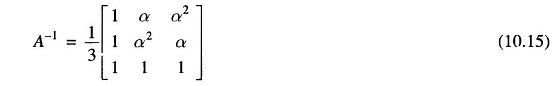In expanded form we can write Eq. (10.14) as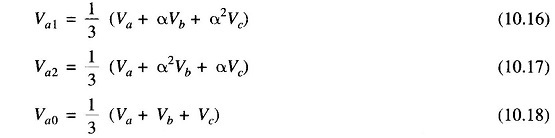Equations (10.16) to (10.18) give the necessary relationships for obtaining symmetrical components of the original phasors, while Eqs. (10.5) to (10.7) give the relationships for obtaining original phasors from the symmetrical components.

The symmetrical component transformation though given above in terms of voltages hold for any set of phasors and therefore automatically apply for a set of currents. Thus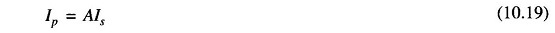and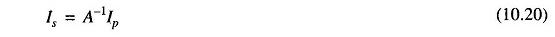where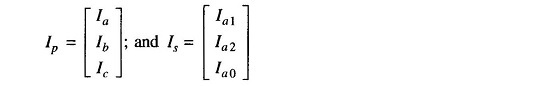Of course A and A-1 are the same as given earlier.

In expanded form the relations (10.19) and (10.20) can be expressed as follows:

(i) Construction of current phasors from their symmetrical components: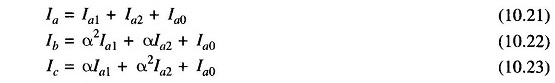(ii) Obtaining symmetrical components of current phasors: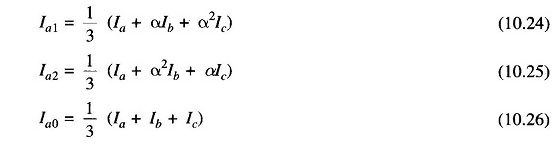Certain observations can now be made regarding a three-phase system with neutral return as shown in Fig. 10.2.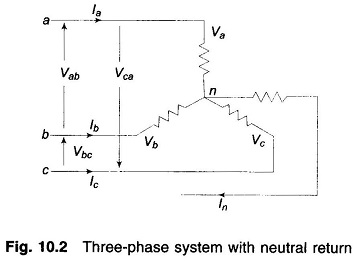The sum of the three line voltages will always be zero. Therefore, the zero sequence component of line voltages is always zero, i.e.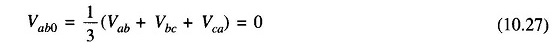On the other hand, the sum of phase voltages (line to neutral) may not be zero so that their zero sequence component Va0 may exist.

Since the sum of the three line currents equals the current in the neutral wire, we have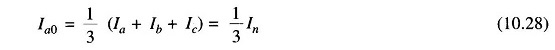i.e. the current in the neutral is three times the zero sequence line current. If the neutral connection is severed,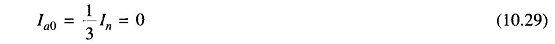i.e. in the absence of a neutral connection the zero sequence line current is always zero.

### Power Invariance:

We shall now show that the symmetrical component transformation is power invariant, which means that the sum of powers of the three symmetrical components equals the three-phase power.

Total complex power in a three-phase circuit is given by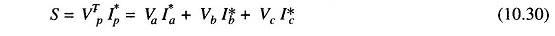or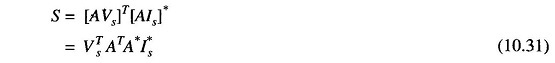Now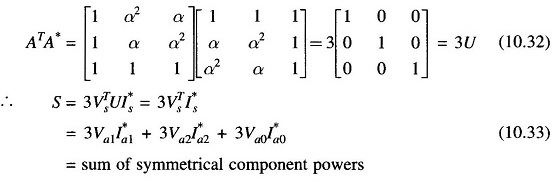Scroll to Top